# Yaskawa Analog Monitor

## Sigma 7 Pulse and Direction Drive

#### Wiring

Wire the analog input to the analog monitors on CN5.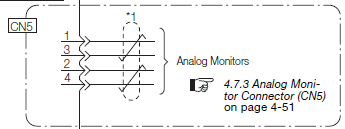Use a cable as shown below: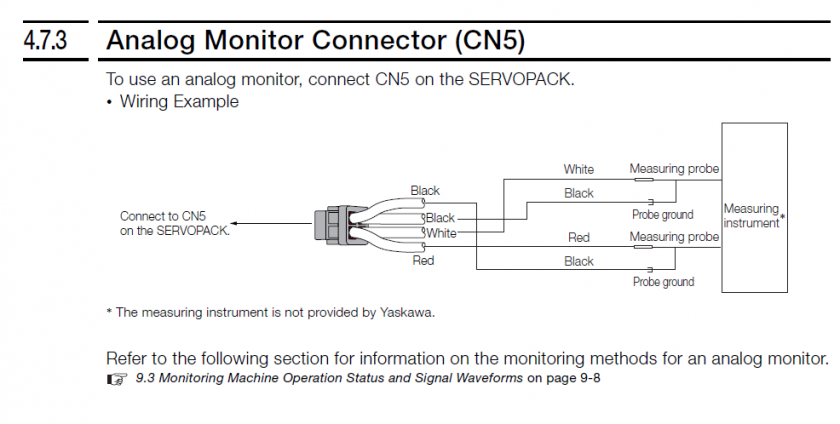#### Setup Options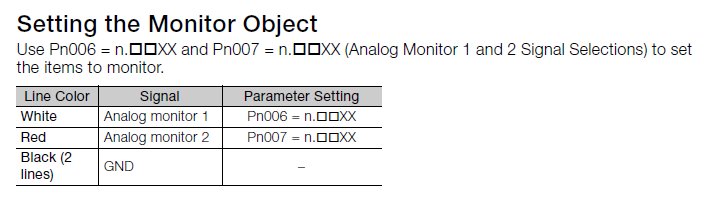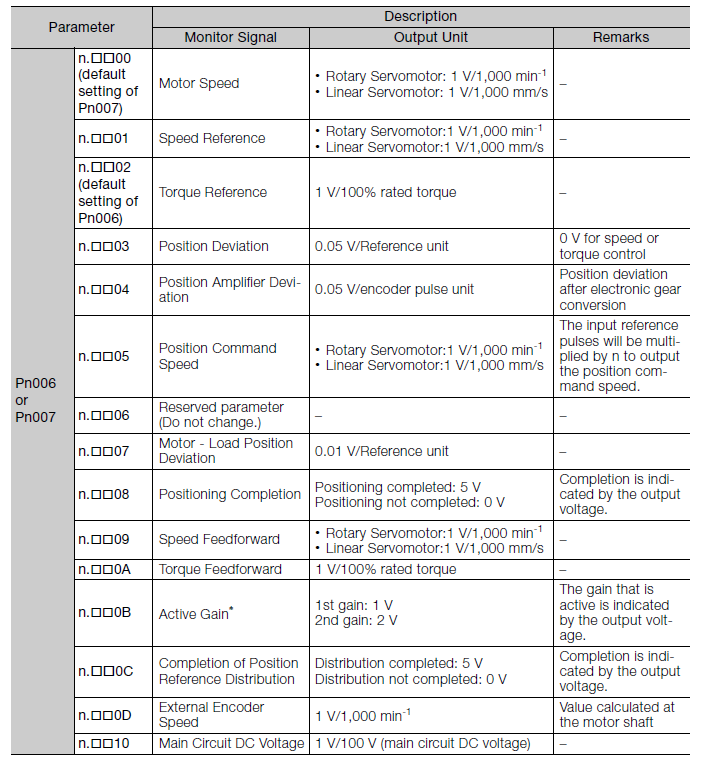Factor and Offset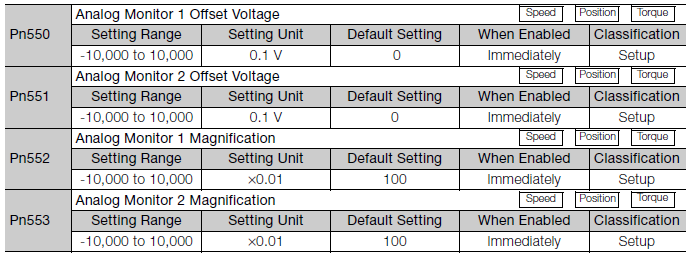#### Load Meter

Analog Monitor 1

Pn006=.XX02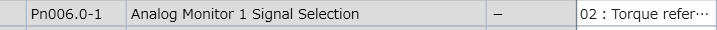Calculate the value of Pn552 based on the max rated torque you will ever see on this device:

Pn552 = 10V / (0.01 * Max Rated Torque %)

 Max Rated Torque % Pn552 40% 2500 50% 2000 100% 1000 300% 333

For example, if you want to see up to 50% of rated torque, calculate it as follows:

Pn552 = 10V / (0.01 * 50%) = 2000 so you would set Pn552 to 2000.

Proof:

Actual Output Voltage = Pn552*0.01 * Actual Rated Torque %

Substitute Max Output Voltage for Actual Output Voltage and Max Torque for Actual Rated Torque:

Max Output Voltage = Pn552*0.01 * Max Rated Torque %

Solve for Pn552:

Pn552 = Max Output Voltage / (0.01 * Max Rated Torque %)

Set Max Output Voltage to 10V:

Pn552 = 10V / (0.01 * Max Rated Torque %)

#### Mach4 Setup

If using the Siemens PLC, see this document: https://support.machmotion.com/books/knowledge-base/page/siemens-plc---wiring-sm1234-analog-card .

Reference Manual: See attached Sigma-7 Manual Analog-Pulse.pdf